Livestock Research for Rural Development 18 (5) 2006 Guidelines to authors LRRD News Citation of this paper

# Comparison between the shapes of lactation curve of Egyptian buffalo milk yield estimated by the incomplete gamma function and a new model

## M A Aziz, N A Shalaby1, O M El-Shafie2, A T Mahdy and A Nishida3

### Department of Animal Production, Faculty of Agriculture, Alexandria University, Alexandria, EgyptFassad9@yahoo.com1Department of Animal Production, Faculty of Agriculture, Al-Mansoura University, Al-Mansoura, Egypt2Animal Research Institute, Ministry of Agriculture, Cairo, Egypt3Laboratory of Animal Breeding and Genetics, Graduate School of Agricultural Science, Faculty of Agriculture, Tohoku University 981-8555 Amamiya-machi, Tsutsumi-dohri, Aoba-ku, Sendai-shi, Japan.

#### Abstract

Weekly milk yield records representing the first ten lactations of 1030 Egyptian buffaloes were used to estimate lactation curve parameters by the nonlinear form of the incomplete gamma function (INGF) and a new nonlinear formula (Yn=b(exp(-n/c)-exp(-n/ac)), and to compare the shape of lactation curves resulted from fitting both models.

The initial yield (a) estimated by INGF ranged between 29.9 kg (the first parity) and 50.2 kg (the tenth parity). The rate of increase to peak production (b) ranged between 0.06 kg (the tenth parity) and 0.23 kg (the first parity), while the rate of decline after peak (c) was fairly constant across parities and ranged between 0.02 and 0.03 kg. On the other hand, fitting the new formula resulted in a rate to reach peak production (a) ranging between 0.002 and 0.024 kg. The maximum milk yield (b) ranged between 44.82 kg (the first parity) and 62.04 kg (the ninth parity) and the rate of changes after maximum yield (c) ranged between 48.32 kg (the first parity) and 86.21 kg (the second parity). The incomplete gamma function underestimated peak yield, while the new model slightly overestimated it. Several criteria were used to compare the results obtained, i.e. weeks to peak production, peak production, total milk yield, t-test for difference between the predicted and actual milk yield and the error mean square.

Generally, the new model gave good fitting to the data and was comparable to the incomplete gamma function.

Key words: Egyptian buffalo, gamma function, lactation curve, milk yield, new model

#### Introduction

Researchers have characterized milk production using a wide variety of statistically estimated equations that regress milk yield per unit time of cows in a given parity against time (Wood 1967, 1969; Nelder 1966, Grossmann and Koops 1988, Rook et al 1993). Although the mathematical formulations of these equations differ, they each produce a similar graph reflecting generally desirable biological characteristics. These characteristics are that milk yield per time unit be peaked and skewed to the right as lactation progresses.

The wide variety of mathematical equations found in the literature can be regarded as alternatives in the sense that each fits some data sets more closely than the other. The form of the fitted curve must be sufficiently flexible to follow closely any trends in the data and to give a consistently good fit to the data. Nevertheless, the incomplete gamma function proposed by Wood (1967) is the most widely used formula for describing lactation curve, however it is criticized for estimating milk production at calving time to be 0 (Scott et al 1996).

A new model for describing lactation curves has been proposed by Hayashi et al (1986), in analogy to the model describing vibration of material point in physics. This model has not been used widely in the study of lactations. However, it is well suited to describe the entire lactation curve. The objectives of this study were to estimate lactation curve parameters using the nonlinear form of the incomplete gamma function and the new model, and to compare the results obtained by both using a data set of weekly milk yield of Egyptian buffaloes.

#### Material and methods

Data on weekly milk yield records representing the first ten lactations of 1030 Egyptian buffaloes were collected from a herd belonging to the Animal Production Research Institute (APRI), the Ministry of Agriculture, Egypt, during the period from 1985 to 1995. The herd is located in Mahelet Mousa, Kafr El-Sheikh governorate, in the Delta region. Numbers of records in the ten parities were 318, 337, 305, 297, 290, 240, 228, 174, 134 and 102, respectively. Animals were given Egyptian clover (Trifolium alexandrinum) in winter and spring, while rice straw was available ad lib in summer and autumn. In addition, they were offered concentrate mixture, all year round, twice daily before milking according to their body weight and milk production. Females were naturally mated and milking was twice daily by hand. Weekly milk yield was the sum of the recorded daily milk production throughout the week. Buffaloes with 10 weeks of milk or less were excluded from the analysis. Maximum number of weeks in milk was 53. Data were adjusted for the effects of year and season of calving and age at calving within parity prior to analysis. Analysis was conducted for each parity, separately. Lactation curves were described by the following formulas:

1. The incomplete gamma function, as suggested by Wood (1967):

Yn=anbexp-cn

Where

Yn is milk yield in week n,
a is a constant representing the level of initial yield of the buffalo cow,
b is a parameter representing the rate of increase to peak,
c is the rate of decline after peak
n is the time period (week).

2. The new model as described by Hayashi et al (1986):

Yn=b(exp-n/c-exp-n/ac)

Where

Yn is milk yield in week n,
a is a parameter related to the rate to reach peak production,
b is a parameter related to the maximum milk yield,
c is a parameter related to the curve changes after maximum yield,
n is time period (week)

Both models were fitted by the Newton method using PROC NLIN of SAS (2000). Starting grids were specified such that all solutions fell within the outer limits of the search grids. Time to peak, maximum yield and total yield over the whole period of lactation were calculated and compared with statistics obtained from the actual data.

The aforementioned parameters were calculated according to the following formulas:

The aforementioned parameters were calculated according to the following formulas:

1.       The incomplete gamma function (Wood, 1967):

a.       Time to peak (nmax) = b/c

b.      Peak Production (ymax)= a(b/c)be-cn  (n is time to peak yield)

c.       Total milk yield=a/cb+1

2.       The new model (Hayashi, et al., (1986):

a.       Time to peak (nmax)= -aclog(a)/(1-a)

b.      Peak Production (ymax)=b(1-a)aa/(1-a)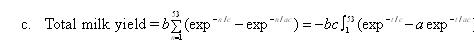Least squares mean of differences between predicted and actual weekly milk yield of each parity was computed and compared using t-test for difference with respect to zero. Errors mean squares resulted from fitting both models were also compared.

#### Results

The parameter estimates and their approximate standard errors calculated by the incomplete gamma function and the new model are shown in Table 1.

 Table 1.  Lactation curve parameters (kg) estimated by the new model and the incomplete gamma function for the ten parities Parity number The new model The incomplete gamma function a b c R2,% a b c R2, % 1 2 3 4 5 6 7 8 9 10 0.024** (0.004)+ 0.01 (0.001) ** 0.016 (0.001) 0.017** (0.001) 0.018** (0.002) 0.013** (0.001) 0.019** (0.002) 0.014** (0.001) 0.012** (0.002) 0.002** (0.001) 44.82** (1.13) 52.06** (0.63) 56.00** (0.66) 56.46** (0.58) 60.26** (0.71) 57.64** (0.66) 61.40** (0.78) 61.60** (0.76) 62.04** (1.04) 54.43** (0.94) 48.32** (2.30) 86.21** (3.25) 63.45** (1.81) 61.98** (1.49) 58.00** (1.52) 67.75** (2.00) 56.26** (1.56) 62.92** (1.86) 69.11** (3.01) 76.65** (3.97) 0.92 0.94 0.97 0.97 0.97 0.96 0.97 0.96 0.92 0.89 29.92** (1.10) 41.79** (1.16) 41.89** (1.00) 41.50** (0.94) 43.84** (0.91) 47.57** (1.41) 44.13** (1.02) 46.97** (0.78) 49.23** (1.52) 50.16** (1.79) 0.23** (0.02) 0.12** (0.02) 0.16** (0.01) 0.17** (0.01) 0.18** (0.01) 0.10** (0.02) 0.18** (0.01) 0.15** (0.01) 0.13** (0.02) 0.06** (0.02) 0.03** (0.001) 0.02** (0.001) 0.02** (0.001) 0.02** (0.001) 0.03** (0.001) 0.02** (0.001) 0.03** (0.001) 0.02** (0.001) 0.02** (0.001) 0.02** (0.001) 0.96 0.93 0.97 0.97 0.98 0.94 0.98 0.98 0.94 0.90 The incomplete gamma function: a is a constant representing the level of initial yield of the buffalo cow, b is a parameter representing the rate of increase to peak and c is the rate of decline after peak. The new model: a is a parameter related to the rate to reach peak production, b is a parameter related to the maximum milk yield and c is a parameter related to the rate of change after maximum yield.  ** Significant at <0.01 + Standard error R2 is the coefficient of determination.

The parameter estimates were all statistically significant (P<0.01), based on the asymptotic standard errors. The estimates fluctuated across parities with no certain trend. Regarding the incomplete gamma function, the highest value of the initial milk yield (a) was in parity 10, while the lowest value was in the first parity. In contrast, the highest rate of increase to peak production (b) was in the first parity and the lowest was in parity 10. The rate of decline after peak (c) was fairly constant and ranged between 0.02 and 0.03 kg. Values of R2 ranged between 0.90 and 0.98. On the other hand, the highest rate to peak production (a) estimated by the new function was in the first parity and the lowest was in parity 10. The maximum milk production (b) ranged between 44.82 kg (the first parity) and 62.04 kg (parity 9). The rate of changes after maximum yield (c) ranged between 48.32 kg (the first parity) and 86.21 kg (the second parity). R2 values ranged between 0.89 and 0.97, and they are approximately equal to the corresponding values obtained by Wood's function. The R2 values are high because the analysis was conducted on the adjusted means rather than individual observations.

In the first parity, the incomplete gamma function slightly underestimated milk yield in the periods between the second and fifth weeks and between week 17 and week 34, then it overestimated actual milk yield up to week 48, and finally underestimated it up to the end of lactation, (Figure 1a). On the other hand, the new model overestimated peak yield up to week 13, followed by an underestimation period up to week 33, which was compensated by an overestimation period thereafter (Figure 1a).Figure 1a. Lactation curve as described by the incomplete gamma function (Wood model) and the new model
in comparison with the actual milk yield of the Egyptian buffaloes in the first parity

Both models perfectly predicted the actual milk yield of the fifth parity, but Wood's function slightly underestimated peak yield and the new model overestimated it (Figure 1b).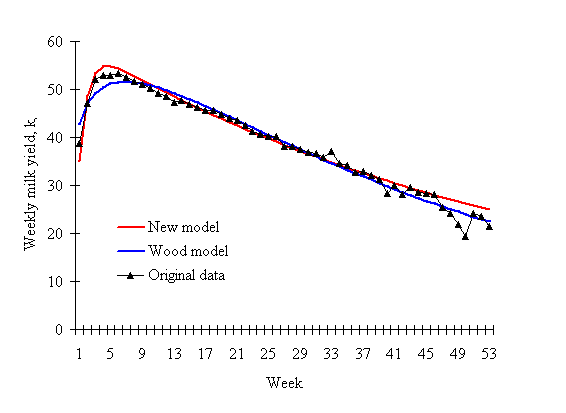Figure 1b. Lactation curve as described by the incomplete gamma function (Wood model) and the new model
in comparison with the actual milk yield of the Egyptian buffaloes in the fifth parity

In parity 10, the lactation curves estimated by both functions were more or less flat. Both models gave higher initial production (overprediction) and flatter peaks (under-prediction), (Figure 1c). This could be due to shifting the model further on the hyperbolic tangent curve because milk yield declines linearly after approximately 7 weeks in milk.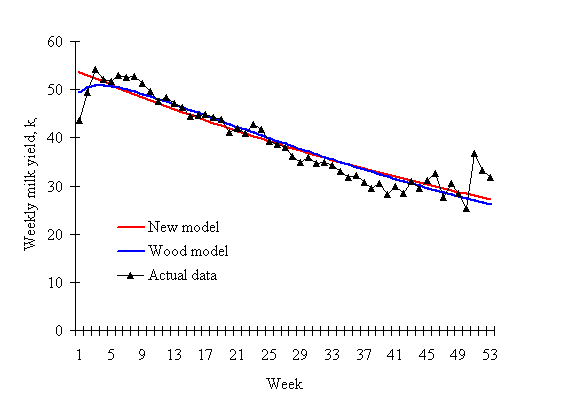Figure 1c. Lactation curve as described by the incomplete gamma function (Wood model) and the new model
in comparison with the actual milk yield of the Egyptian buffaloes in the tenth parity

Several workers have estimated lactation curve parameters of Egyptian buffaloes. Samak et al (1988) described lactation curve of milk yield of Egyptian buffaloes using the linear form of the incomplete gamma function and reported values of the initial milk yield (a) ranging between 17.43 and 27.43 kg in the first and fourth parities, respectively. These values were lower than those reported in the present study. The rate of increase to peak production (b) and the rate of decline after peak (c) in their study ranged between 0.421 and 0.537 kg and between 0.04 and 0.05 kg, respectively and they were higher than the corresponding values found in the present study. Ibrahim (1995) estimated lactation curve parameters for milk yield of Egyptian buffaloes using the nonlinear form of the incomplete gamma function. The initial milk yield (a) in his study ranged between 37.20 and 52.90 kg and the estimates of b and c were between 0.31 and 0.37 kg and between 0.06 and 0.08 kg, respectively. Sadek, el al., (1998) reported similar results to those obtained by Ibrahim (1995), ranging between 35.5 and 52.3 kg for the initial milk yield (a), between 0.27 and 0.36 kg for the rate of increase to peak production (b) and between 0.05 and 0.07 kg for the rate of decline after peak (c). The results obtained in both studies are higher than those reported in the present study. Aziz et al (2003), reported values of the initial milk yield (a) for the first six lactations, ranging between 30.30 and 44.38 kg. The rate of increase to peak production (b) in their study ranged between 0.17 and 0.27 kg and those of the rate of decrease after peak (c) were between 0.02 and 0.03 kg. In their study, the initial milk yield (a) increased with increasing parity, while the rate of increase to peak production (b) decreased which is not in accordance with the findings of the present study. However, the estimates of the present study are in close agreement with those reported in their study. It is not possible to compare the results of the new formula with other studies, as there is no information found in the literature on this function.

The relative performance and the predictive ability of the new model should be computed and compared with the corresponding values estimated by the incomplete gamma function and the actual data. Table 2 provides weeks to peak production and maximum milk production estimated by both functions and the actual data.

 Table 2.  Weeks to peak yield and peak yield (kg) estimated by the new model and the incomplete gamma function in comparison with the actual yield for the ten parities Parity number The actual milk yield The new model The incomplete gamma function Week Peak yield Week Peak yield Week Peak yield 1 2 3 4 5 6 7 8 9 10 4.00 4.00 4.00 5.00 6.00 6.00 4.00 6.00 5.00 3.00 37.67 48.97 50.22 50.73 53.25 54.06 53.79 54.65 56.46 54.15 4.47 4.00 4.22 4.39 4.33 3.83 4.39 3.86 3.69 1.13 39.87 49.20 51.57 51.70 54.91 53.78 55.69 57.11 58.11 53.50 7.11 6.94 6.82 6.94 6.82 5.22 6.84 6.53 6.26 3.51 37.39 46.74 48.57 48.62 51.57 50.84 52.27 53.80 54.92 50.92

Weeks to peak production estimated by the new model ranged between 1.13 and 4.47 weeks, while the corresponding values estimated by the gamma function were between 3.51 and 7.11 weeks. The range observed for the actual data was between 3 and 6 weeks. Weeks to peak production estimated by the new model were lower than those of the actual data, with some exceptions viz. week to peak production of the second parity was equal to that of the actual data, while the values observed in the first, third and seventh parities were higher than those of the actual data. The corresponding values estimated by the incomplete gamma function were generally higher than those of the actual data, except that of the sixth parity which it was lower.

Peak yields estimated by the new model were slightly higher than the corresponding values of the actual data, except those of the sixth and tenth parities where they were approximately equal. The estimates obtained by the incomplete gamma function were lower than those of the actual data, except that of the first parity which it was equal. Peak yield estimated by both functions increased with increasing parity up to the fifth. The lowest peak production was in the first parity for both models and the highest was in the ninth parity.

Total milk yield, t-test for the difference between the actual data and the predicted values, and the residual mean squares are presented in Table 3.

 Table 3.  Residual mean squares, t-test for difference between actual and predicted values and total milk yield of the ten parities as estimated by the new model (N) and the incomplete gamma function (W) Parity number Residual mean squares t-test for difference Total milk yield W N W N W N Actual 1 2 3 4 5 6 7 8 9 10 2.90 3.29 2.43 2.20 2.05 4.70 2.57 1.46 5.47 7.25 5.43 2.79 2.56 1.87 2.73 2.75 3.19 3.43 6.85 8.05 0.01 0.13 0.02 -0.17 0.01 -1.91+ -0.08 0.05 0.12 -0.11 0.08 0.00 0.03 0.06 2.22* -0.08 0.06 0.07 0.02 0.00 1393 2027 1963 1957 2036 2040 2046 2158 2255 2066 1395 2025 1964 1959 2063 2077 2048 2159 2253 2068 1393 2025 1963 1959 2036 2078 2047 2158 2253 2068 * Significant at P<0.05 + Significant at P<0.10

Total milk yields estimated by the new model were approximately equal to the actual total milk yields, except that of the fifth parity where the predicted value was higher. On the other hand, the incomplete gamma function tended to slightly underestimate or overestimate the actual milk yield. Values of t-test for least squares means of differences were not significantly different from zero, except that of the fifth parity in the case of the new model and sixth parity in the case of the incomplete gamma function.

Residuals means squares resulted from fitting the incomplete gamma function of the first and the fifth parities as well as parities 7 to 10 were lower than those resulted from fitting the new model. The differences between the actual and predicted weekly milk yields for both models are plotted in Figures 2 (a and b) for the ten parities.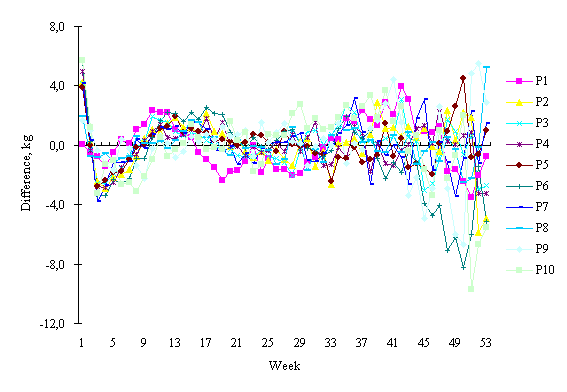Figure 2a. The differences between the actual and the predicted values resulted from fitting the incomplete gamma function for the ten parities.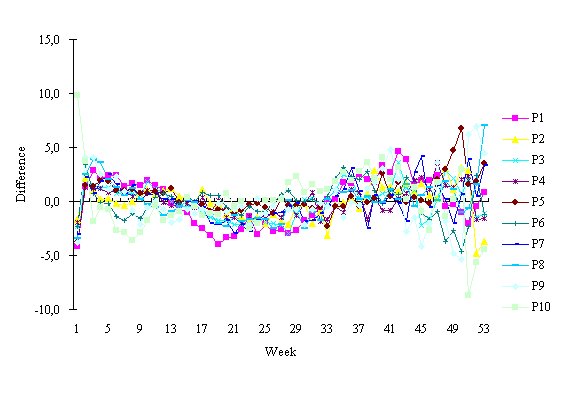Figure 2b. The differences between the actual and the predicted values of milk yield resulted from fitting the new model for the ten parities.

For the fitted curve to be an adequate representation of the data, the residuals should show no evidence of trends but should be randomly scattered about the horizontal axis. From the figures, it could be seen that the new model overestimated early yield up to peak production, while the incomplete gamma function underestimated it. As the lactation progressed, both functions behaved in a similar manner.

#### Discussion

The results indicated that the new model could be as robust as the incomplete gamma function for describing lactation curve parameters and perform well overall with respect to the underlying process, in that it behaves as the physiological condition it is trying to represent. In some instances, the new model performed better than Wood's function. One possible explanation for the poor performance of both models in some parities could be attributed to the high variability in milk yield in these parity groups, as reflected by high residual mean squares (Table 3). Several other algorithms were also tried in order to improve the performance of both equations, but the results were similar. The performance of both equations across parities was fairly uniform, i.e. the incomplete gamma function underestimated peak yield, while the new model overestimated it. As lactation progressed, the lactation curve became nearly linear and the equations could readily predict this linearity rather than the nonlinear prevailed in early lactation up to peak. The estimation process was, in fact, not without problems, i.e. the parameters estimated in some parities may not be estimated precisely due to the high variability in milk yield, resulting in a detrimental effect on locating the maximum point on the marginal likelihood surface. For example, lactation curve in the tenth parity was more or less flat, which is an unrealistic. In this parity group, R2 values estimated by both models were the lowest (Table 1). The maximum likelihood methods of solving both equations are more difficult to compute, although they give better results. They are based on the iterative process and they must converge.

The new model presented here is purely empirical and needs further investigation in relation to the physiological processes involved in lactation. An analytical and empirical comparison of various nonlinear models with the new model may also be worth investigated. The behavior of the new model with particular reference to the effect of parity and seasonality needs investigation. It is also worthy to determine an equation for persistency of lactation based on this model. The usefulness of the new model in providing precise measurements of milk yield in relation to treatment changes in feeding trials, accurate estimates of total milk yield from incomplete records and reliable forecast of stage by stage for individual or group of dairy animals should be investigated.

#### References

Aziz M A, Mahdy A E, El-Shafie O M and Shalaby N A 2003 A comparison of different models of the lactation curve in Egyptian buffaloes. Journal of Agricultural Science, Mansoura University 28(7): 5253-5268.

Grossman M and Koops W J 1988 Multiphasic analysis of lactation curves in dairy cattle. Journal of Dairy Science 71: 1598-1608.

Hayashi T, Nagamine Y and Nishida A 1986 A vibration model to describe the lactation curve of a dairy cow. Japanese Journal of  Zootechnical Science 57: 471-478.

Ibrahim M A M 1995 The use of Gamma-type function in describing the lactation curve of Egyptian buffaloes. Egyptian Journal of Animal Production 32: 113-123.

Nelder J A 1966 Inverse-polynomial, a useful group of multifactor response functions. Biometrics 22: 128-141.

Rook A J, France F and Dhanoa M S 1993 On the mathematical description of lactation curves. Journal of Agriculture Science, Cambridge 121: 97-102.

Sadek R R, Mohamed M M, Ibrahim M A M and Abdel-Lattef  H M A 1998 Estimation of lactation curve parameters in Egyptian buffaloes. Egyptian Journal of Animal Production 35: 1-27.

Samak M A, Hassan G A, Hassan A and Yassen N 1988  The lactation curve and performance of the Egyptian buffalo. Alexandria Science Exchange 9: 83-97.

SAS. 2000 SAS Institute Inc. SAS User's Guide, Statistics. Cary, NC.

Scott T A, Yandell B, Zepeda L, Shaver R D and Smith T R 1996 Use of lactation curve for analysis of milk production data. Journal of Dairy Science 79: 1885-1894. http://jds.fass.org/cgi/reprint/79/10/1885

Wood P D P 1967 Algebraic model of the lactation curve in cattle. Nature 216: 164-165.

Wood P D P 1969 Factors affecting the shape of the lactation curve in cattle. Animal Production 11: 307-316.

Received 9 October 2005; Accepted 2 March 2006; Published 11 May 2006

Go to top